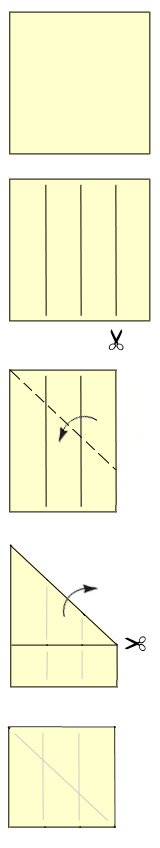# Divide Paper for Origami

To fold or divide paper accurately into sections is something that you will need to do when you progress to the intermediate and more advanced origami models. To divide a sheet of paper in half, quarters, eighths, sixteenths etc. is fairly easy: simply use the edges of the paper to fold the sheet in half, then continue to fold each section in half to get smaller and smaller divisions.

However, dividing paper into thirds, fifths, sevenths etc can be challenging. There are many ways to divide a sheet of paper, some methods are easier than others. The method shown here is:
– fairly easy,
– requires scissors, and
– generates a piece of paper smaller than the original sheet.#### Divide Paper: easy method using scissors

• Take a sheet of paper and fold it into a convenient number of sections which is greater than the number of sections you need.

• For example, if you want to divide your paper into thirds, then fold the paper into quarters.

• Use scissors to cut off one of the sections.

• Fold the paper as shown and then cut off the excess.

• You will get a square sheet of paper which is divided into thirds. The paper is a little smaller than the original sheet of paper, but it is square and it is accurately divided into thirds.

• The same can be done with larger divisions: fold the paper into sixths then cut off one section to get five divisions. Fold the paper into eights to get 7 divisions. So forth.

• This method of dividing paper is not particularly elegant, but it is easy and gets the job done. It can be used only when it is the first step in making the origami model and you can cut the paper. This method cannot be used when you are in the middle of folding an origami model and cutting the paper is not an option.

• See some of the other methods of dividing paper into equal segments.